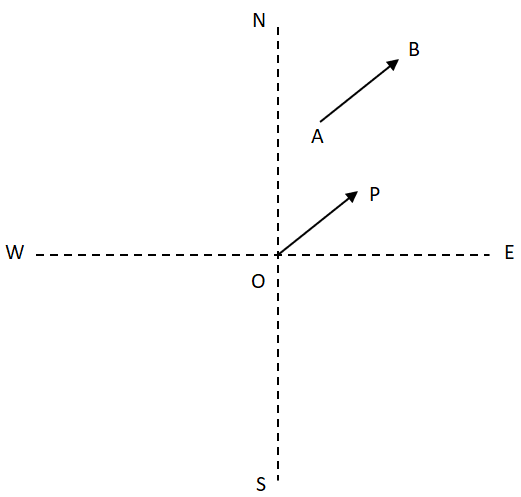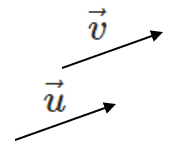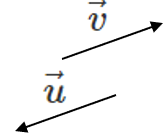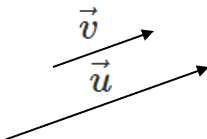# Properties of Vectors

A Position Vector is a vector whose tail is moved to the origin. Remember that vectors can change position (where they are in space) as long as they have the same magnitude and direction (Equal Vectors).

Here, vector $$\vec{AB} = \vec{OP}$$, i.e., they are Equivalent Vectors. Point $$O$$ is the origin. It is convienent to move vectors to the origin because if point is $$P(3,2)$$ we know the x and y components so $$\vec{OP} = \left<3,2\right>$$.Otherwise, a position vector $$\vec{OP}$$ can be found from points $$A(a_x,a_y)$$ and $$B(b_x,b_y)$$:

$$\vec{OP} = \vec{AB} = \left< b_x - a_x, b_y - a_y \right>$$

Vectors differ from lines because they have starting and end points and they can be moved around.

Equivalent or Equal Vectors have the same magnitude and direction but do not need to be in the same position:

$$\vec{u}=\vec{v}$$Negative or Opposite Vectors have the same magnitude but opposite directions:

$$\vec{u}=-\vec{v}$$

$$|\vec{u}|=|\vec{v}|$$If $$A$$ and $$B$$ are points in space then $$\vec{AB} =-\vec{BA}$$

The Zero Vector has a magnitude of 0 and no specified direction like $$\vec{0}$$, $$\textbf{0}$$ or $$\left<0,0,0\right>$$.

##### Try these questions:

Collinear or Parallel Vectors have the same direction but different magnitudes. For example, $$\vec{u}=\left<1,1,1\right>$$ and $$\vec{v}=\left<2,2,2\right>$$. Since the vectors are in the same direction, we just need to stretch (or compress) one out to make it equal to the other:

$$\vec{v}=\frac{1}{2}\vec{u}$$Multiplying a vector by a scalar results in a new vector that has a different magnitude but same direction.

Vectors can also be parallel if they are in opposite directions by multiplying by a negative scalar.

##### Try these questions:

The table below summarizes some properties of vectors:

 $$\vec{u} + \vec{0} = \vec{u}$$ $$\vec{u} + (-\vec{u}) = \vec{0}$$ $$c (\vec{u} + \vec{v}) = c \vec{u} + c \vec{v}$$ $$(c + d) \vec{u} = c \vec{u} + d \vec{u}$$ $$(cd) \vec{u} = c (d \vec{u})$$ $$1 \vec{u} = \vec{u}$$